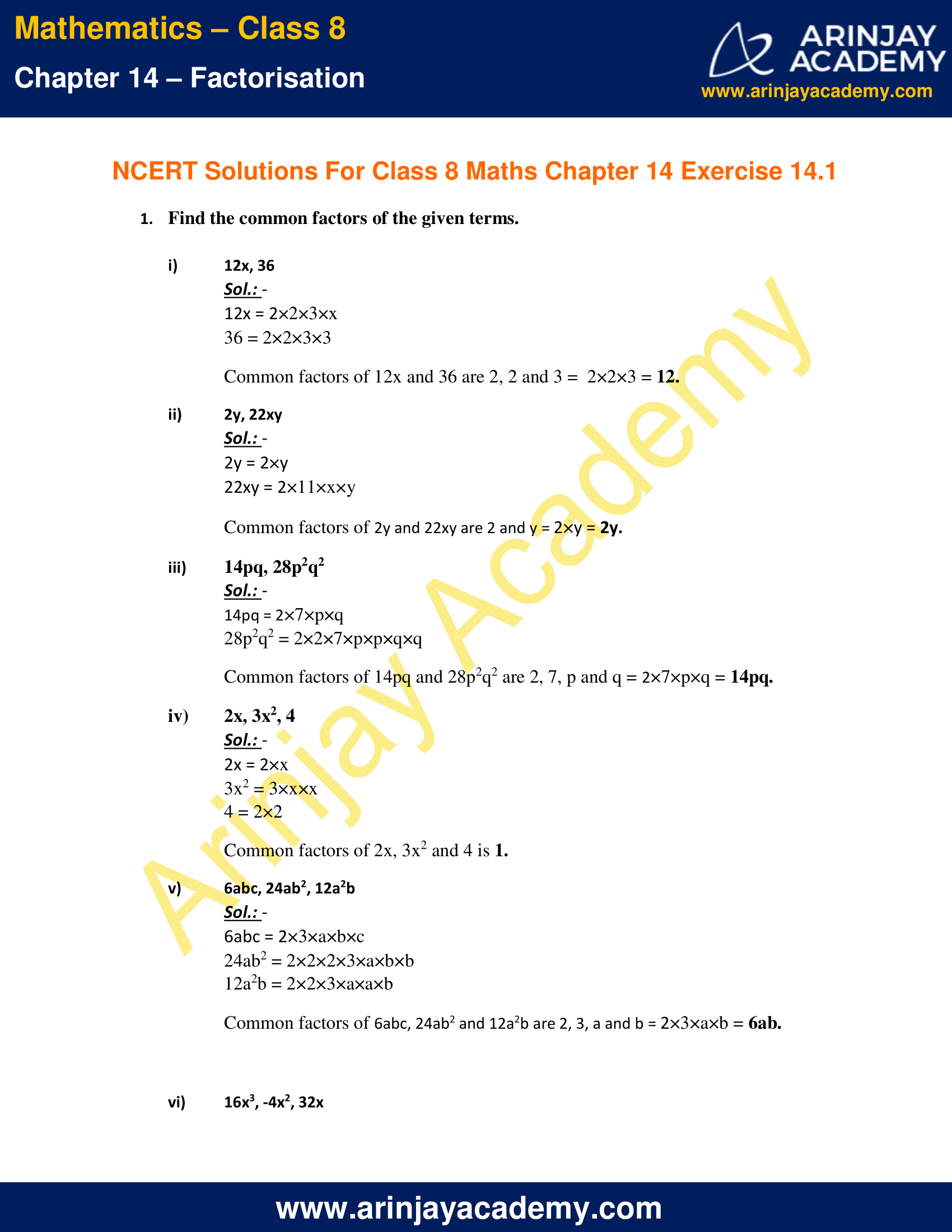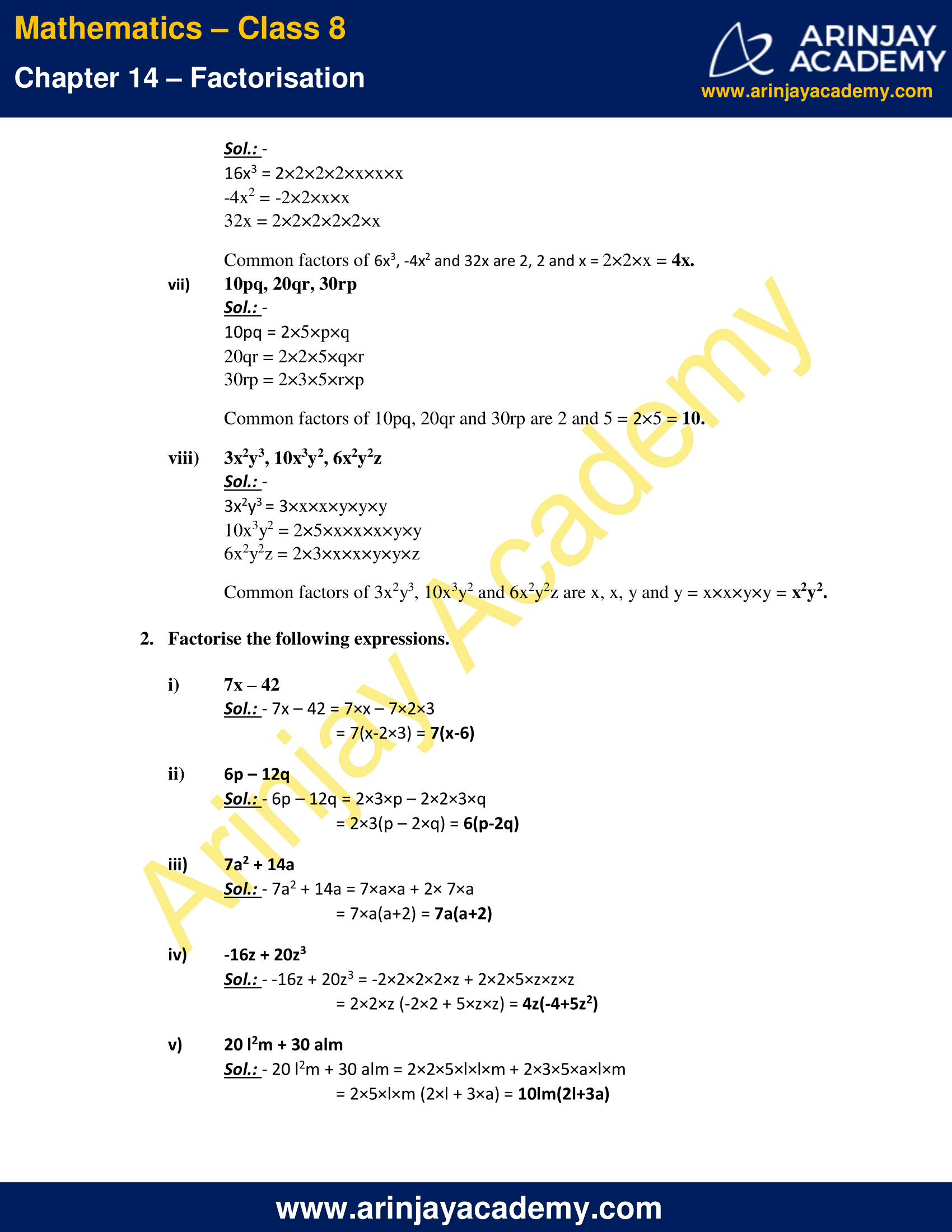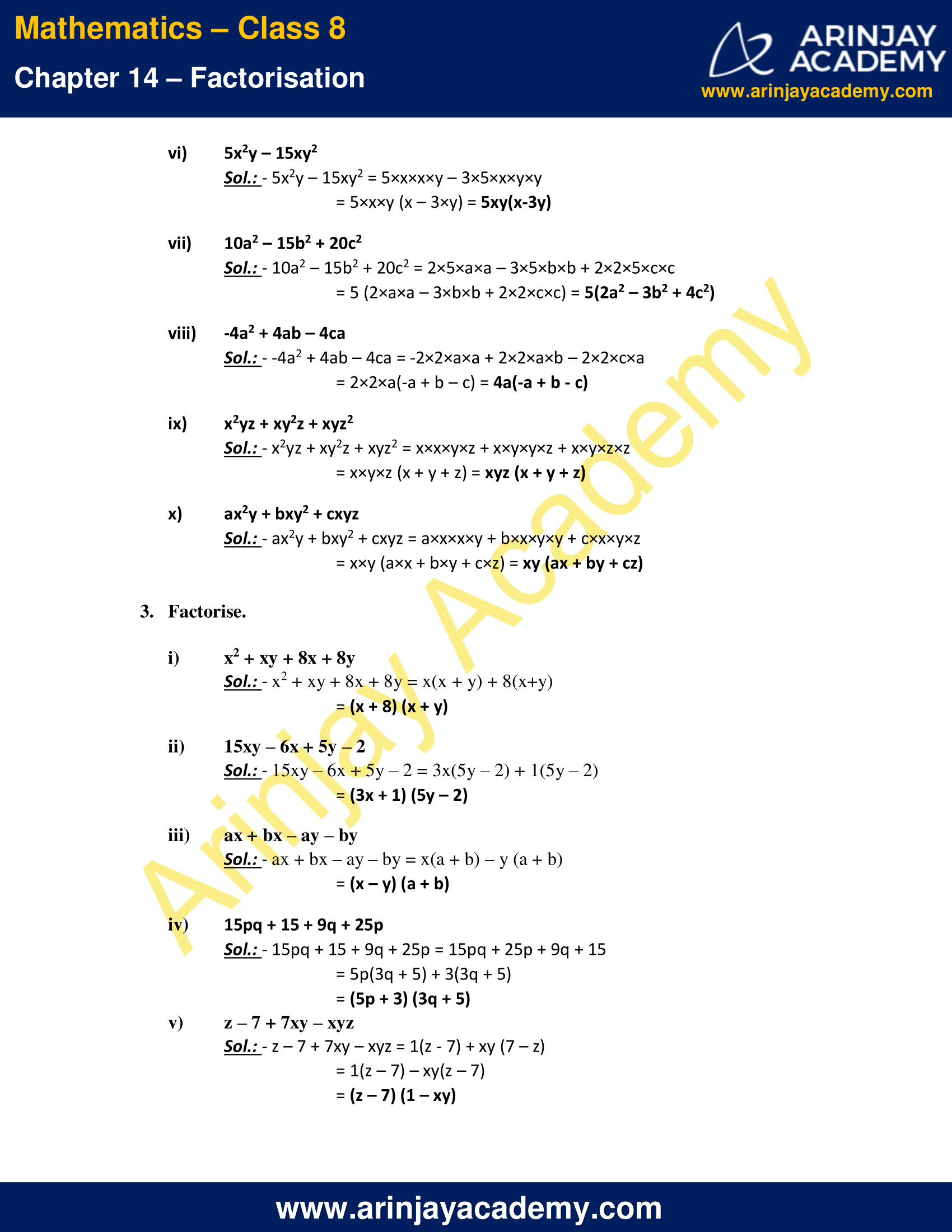# NCERT Solutions for Class 8 Maths Chapter 14 Exercise 14.1 – Factorisation

NCERT Solutions for Class 8 Maths Chapter 14 Exercise 14.1 – Factorisation, has been designed by the NCERT to test the knowledge of the student on the following topics:-

• Introduction
– Factors of natural numbers
– Factors of algebraic expressions
• What is Factorisation?
– Method of common factors
– Factorisation by regrouping terms
• What is regrouping?

### NCERT Solutions for Class 8 Maths Chapter 14 Exercise 14.1 – FactorisationNCERT Solutions for Class 8 Maths Chapter 14 Exercise 14.1 – Factorisation

Find the common factors of the given terms.

i) 12x, 36

Sol.:

12x = 2×2×3×x
36 = 2×2×3×3
Common factors of 12x and 36 are 2, 2 and 3 =  2×2×3 = 12.

ii) 2y, 22xy

Sol.:

2y = 2×y
22xy = 2×11×x×y
Common factors of 2y and 22xy are 2 and y = 2×y = 2y.

iii) 14pq, 28p2q2

Sol.:

14pq = 2×7×p×q
28p2q2 = 2×2×7×p×p×q×q
Common factors of 14pq and 28p2q2 are 2, 7, p and q = 2×7×p×q = 14pq.

iv) 2x, 3x2, 4

Sol.:

2x = 2×x
3x2 = 3×x×x
4 = 2×2
Common factors of 2x, 3x2 and 4 is 1.

v) 6abc, 24ab2, 12a2b

Sol.:

6abc = 2×3×a×b×c
24ab2 = 2×2×2×3×a×b×b
12a2b = 2×2×3×a×a×b
Common factors of 6abc, 24ab2 and 12a2b are 2, 3, a and b = 2×3×a×b = 6ab.

vi) 16x3, -4x2, 32x

Sol.:

16x3 = 2×2×2×2×x×x×x
-4x2 = -2×2×x×x
32x = 2×2×2×2×2×x
Common factors of 6x3, -4x2 and 32x are 2, 2 and x = 2×2×x = 4x.

vii) 10pq, 20qr, 30rp

Sol.:

10pq = 2×5×p×q
20qr = 2×2×5×q×r
30rp = 2×3×5×r×p
Common factors of 10pq, 20qr and 30rp are 2 and 5 = 2×5 = 10.

viii) 3x2y3, 10x3y2, 6x2y2z

Sol.:

3x2y3 = 3×x×x×y×y×y
10x3y2 = 2×5×x×x×x×y×y
6x2y2z = 2×3×x×x×y×y×z
Common factors of 3x2y3, 10x3y2 and 6x2y2z are x, x, y and y = x×x×y×y = x2y2.

2. Factorise the following expressions.

i) 7x – 42

Sol.: – 7x – 42
= 7×x – 7×2×3
= 7(x-2×3)
= 7(x-6)

ii) 6p – 12q

Sol.: – 6p – 12q
= 2×3×p – 2×2×3×q
= 2×3(p – 2×q)
= 6(p-2q)

iii) 7a2 + 14a

Sol.: – 7a2 + 14a
= 7×a×a + 2× 7×a
= 7×a(a+2)
= 7a(a+2)

iv) -16z + 20z3

Sol.: – -16z + 20z3
= -2×2×2×2×z + 2×2×5×z×z×z
= 2×2×z (-2×2 + 5×z×z)
= 4z(-4+5z2)

v) 20 l2m + 30 alm

Sol.: – 20 l2m + 30 alm
= 2×2×5×l×l×m + 2×3×5×a×l×m
= 2×5×l×m (2×l + 3×a)
= 10lm(2l+3a)

vi) 5x2y – 15xy2

Sol.: – 5x2y – 15xy2
= 5×x×x×y – 3×5×x×y×y
= 5×x×y (x – 3×y)
= 5xy(x-3y)

vii) 10a2 – 15b2 + 20c2

Sol.: – 10a2 – 15b2 + 20c2
= 2×5×a×a – 3×5×b×b + 2×2×5×c×c
= 5 (2×a×a – 3×b×b + 2×2×c×c)
= 5(2a2 – 3b2 + 4c2)

viii) -4a2 + 4ab – 4ca

Sol.: – -4a2 + 4ab – 4ca = -2×2×a×a + 2×2×a×b – 2×2×c×a
= 2×2×a(-a + b – c)
= 4a(-a + b – c)

ix) x2yz + xy2z + xyz2

Sol.: – x2yz + xy2z + xyz2
= x×x×y×z + x×y×y×z + x×y×z×z
= x×y×z (x + y + z)
= xyz (x + y + z)

x) ax2y + bxy2 + cxyz

Sol.: – ax2y + bxy2 + cxyz
= a×x×x×y + b×x×y×y + c×x×y×z
= x×y (a×x + b×y + c×z)
= xy (ax + by + cz)

3. Factorise

i) x2 + xy + 8x + 8y

Sol.: – x2 + xy + 8x + 8y
= x(x + y) + 8(x+y)
= (x + 8) (x + y)

ii) 15xy – 6x + 5y – 2

Sol.: – 15xy – 6x + 5y – 2
= 3x(5y – 2) + 1(5y – 2)
= (3x + 1) (5y – 2)

iii) ax + bx – ay – by

Sol.: – ax + bx – ay – by
= x(a + b) – y (a + b)
= (x – y) (a + b)

iv) 15pq + 15 + 9q + 25p

Sol.: – 15pq + 15 + 9q + 25p
= 15pq + 25p + 9q + 15
= 5p(3q + 5) + 3(3q + 5)
= (5p + 3) (3q + 5)

v) z – 7 + 7xy – xyz

Sol.: – z – 7 + 7xy – xyz
= 1(z – 7) + xy (7 – z)
= 1(z – 7) – xy(z – 7)
= (z – 7) (1 – xy)

The next Exercise for NCERT Solutions for Class 8 Maths Chapter 14 Exercise 14.2 – Factorisation can be accessed by clicking here

Download NCERT Solutions for Class 8 Maths Chapter 14 Exercise 14.1 – Factorisation